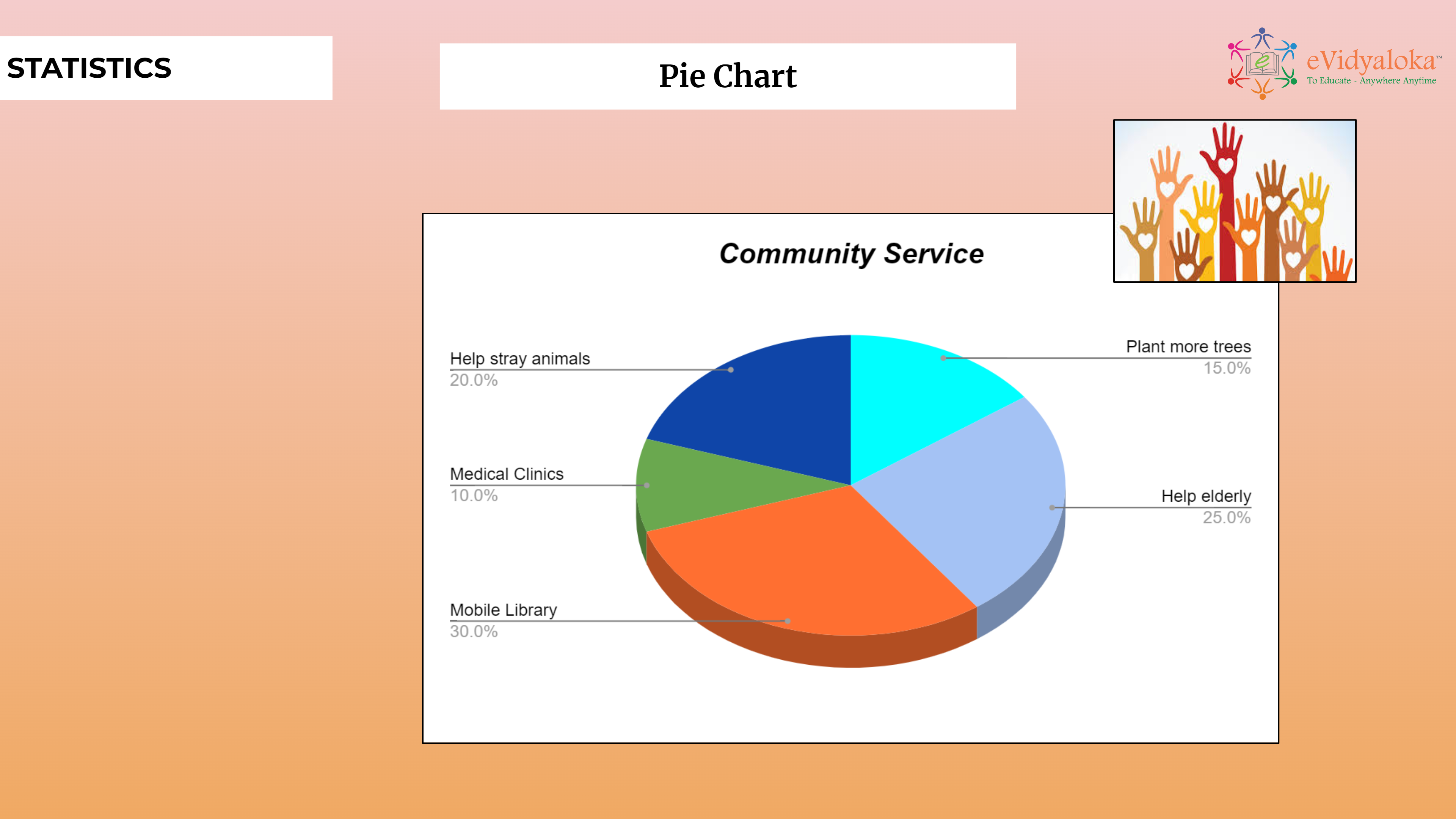# Statistics

``````

Dear Volunteer Teacher,

Kindly teach the bridge concept of Statistics prior to teaching the related textbook chapters.
Thank you.

## Course Content

• Using the Situation-,Community building activities, data collection, prepration of frequency table and representation in bar graph and pie chart and histogram.
• Calculating the % , Converting into angles, Construction of Pie chart-
• Difference between btw Bar grpah and Histogram.
• Definition and construction of a frequency polygon for the same data.

## Learning Outcomes

Learning outcomes has been listed below which will serve as your guide for what students need to learn.

SWBAT:

• Represent trhe given data as a Bar grpah and convert the same into a pie chart.
• The will laos be able to prepare the frequency table and preapre an histogram and frequency polygon.

## PPT Deck

To make the lesson come alive, please find below PPT deck